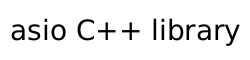### Scheduler concept

```template<class S>
concept scheduler =
copy_constructible<remove_cvref_t<S>> &&
equality_comparable<remove_cvref_t<S>> &&
requires(E&& e) {
execution::schedule((E&&)e);
};
```

None of a scheduler's copy constructor, destructor, equality comparison, or `swap` operation shall exit via an exception.

None of these operations, nor a scheduler type's `schedule` function, or associated query functions shall introduce data races as a result of concurrent invocations of those functions from different threads.

For any two (possibly const) values `x1` and `x2` of some scheduler type `X`, ```x1 == x2``` shall return `true` only if ```asio::query(x1, p) == asio::query(x2, p)``` for every property `p` where both `asio::query(x1, p)` and ```asio::query(x2, p)``` are well-formed and result in a non-void type that is `EqualityComparable` (C++Std [equalitycomparable]). [Note: The above requirements imply that ```x1 == x2``` returns `true` if `x1` and `x2` can be interchanged with identical effects. A scheduler may conceptually contain additional properties which are not exposed by a named property type that can be observed via `asio::query`; in this case, it is up to the concrete scheduler implementation to decide if these properties affect equality. Returning `false` does not necessarily imply that the effects are not identical. —end note]

A scheduler type's destructor shall not block pending completion of any receivers submitted to the sender objects returned from `schedule`. [Note: The ability to wait for completion of submitted function objects may be provided by the execution context that produced the scheduler. —end note]

In addition to the above requirements, type `S` models `scheduler` only if it satisfies the requirements in the Table below.

In the Table below,

• `s` denotes a (possibly const) scheduler object of type `S`,
• `N` denotes a type that models `sender`, and
• `n` denotes a sender object of type `N`

Table 27. Scheduler requirements

expression

return type

operation semantics

`execution::schedule(s)`

`N`

Evaluates `execution::schedule(s)` on the calling thread to create `N`.

`execution::start(o)`, where `o` is the result of a call to `execution::connect(N, r)` for some receiver object `r`, is required to eagerly submit `r` for execution on an execution agent that `s` creates for it. Let `rc` be `r` or an object created by copy or move construction from `r`. The semantic constraints on the `sender` `N` returned from a scheduler `s`'s `schedule` function are as follows:

• If `rc`'s `set_error` function is called in response to a submission error, scheduling error, or other internal error, let `E` be an expression that refers to that error if `set_error(rc, E)` is well-formed; otherwise, let `E` be an `exception_ptr` that refers to that error. [Note: `E` could be the result of calling `current_exception` or `make_exception_ptr`.] The scheduler calls `set_error(rc, E)` on an unspecified weakly-parallel execution agent ([Note: An invocation of `set_error` on a receiver is required to be `noexcept`]), and
• If `rc`'s `set_error` function is called in response to an exception that propagates out of the invocation of `set_value` on `rc`, let `E` be `make_exception_ptr(receiver_invocation_error{})` invoked from within a catch clause that has caught the exception. The executor calls `set_error(rc, E)` on an unspecified weakly-parallel execution agent, and
• A call to `set_done(rc)` is made on an unspecified weakly-parallel execution agent ([Note: An invocation of a receiver's `set_done` function is required to be `noexcept`]).

[Note: The senders returned from a scheduler's `schedule` function have wide discretion when deciding which of the three receiver functions to call upon submission. —end note]